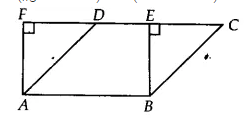Q

# Parallelogram ABCD and rectangle ABEF are on the same base AB and have equal areas. Show that the perimeter of the parallelogram is greater than that of the rectangle.

Q: 1     Parallelogram ABCD and rectangle ABEF are on the same base AB and have equal areas. Show that the perimeter of the parallelogram is greater than that of the rectangle.

ViewsWe have ||gm ABCD and a rectangle ABEF both lie on the same base AB such that, ar(||gm ABCD) = ar(ABEF)
for rectangle,  AB = EF
and for ||gm AB =  CD
CD = EF
AB + CD  =  AB + EF ...........(i)

SInce BEC and  AFD are right angled triangle
Therefore, AD > AF and BC > BE
(BC + AD ) > (AF + BE)...........(ii)

Adding equation (i) and (ii), we get

(AB + CD)+(BC + AD) > (AB + BE) + (AF + BE)
(AB + BC + CD + DA) > (AB + BE + EF + FA)
Hence proved, perimeter of ||gm ABCD is greater than perimeter of rectangle ABEF

Exams
Articles
Questions